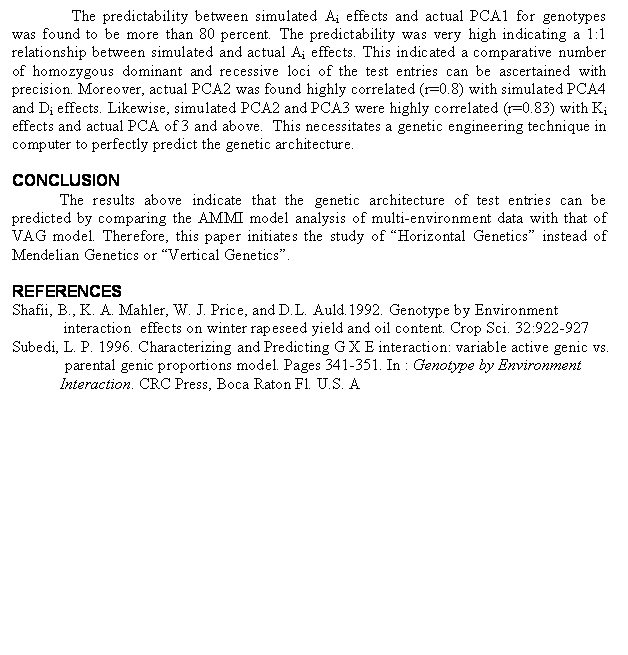## QUANTIFYING AMMI MODEL IN TERMS OF GENETIC PARAMETERS

L. P. Subedi

IAAS/Tribhuvan University, Rampur, Chitwan, Nepal

E-mail:<kiran@scn.mos.com.np>

ABSTRACT

Genotype-by-environment interaction (GEI) is a measure of differential response of genotypes to varied environmental conditions. Its effects are to limit the accuracy of yield estimation from multienvironmental evaluation of varietal performance. There are various models developed by different workers to predict the GEI. I have developed two models: variable active genic (VAG) and variable active parental genic proportions (VAPGeP) model. There are other models like AMMI (additive main effects and multiplicative interactions), that are extensively used these days. Non genetic parameters like eigen value and eigen vectors as well as principal components are computed in the latter model to predict GEI. In this presentation, I have equated different genetic parameters of the genetic model (my model) to eigen value, vectors and principal components and have provided their genetic meaning. For these purpose different populations: purelines and composites were computer generated.  In general, the following relationship was established.

(GxE)ij=(`Ai.-`A..)(aj -`a.)+(Di.-`D..)(dj -`d.) +.. (In terms of genetic model = VAG model)

=±l1v1iu1j  ±l2v2iu2j ±..  (in terms of AMMI model) = Yij -`Yi.-`Y.j +`Y..

Where  Ai= difference between number of homozygous dominant and homozygous recessive loci of ith genotype            Di= Number of heterozygous loci of ith genotype; aj=additive genetic effect for jth environment          dj=dominant  effect  for jth environment      lm=square root of mth eigen value           vmi and umj are corresponding genotypic and environmental eigen vectors.Yij refers to the yield of ith genotype in jth environment. The variances of distribution generating aj’s and dj’s were significantly correlated with eigen values, and Ai and Di effects were highly correlated with genotypic eigen vectors. Similarly, aj and dj effects were found highly correlated with environmental eigen vectors. However, the AMMI model was found mechanical as compared to the VAG model. Moreover, active genetic constitution can also be computed by simulating ANOVA and predicting genetic parameters.

KEYWORD: purelines, composites, eigen value, eigen vector, genetic constitution

INTRODUCTION

Evaluation of genotypic performance in multi environment trials is the main activity in any plant-breeding program. Understanding and predicting of crop response to environment is very important. It is an outcome of the research work in physiology, agronomy, modeling and, genetics and plant breeding. Better understanding of adaptation leads to more efficient and targeted crop improvement strategy. Level of adaptation is a function of the genotype or the genetic constitution and its response to the environment it occupies. In other words, it is the function of the genes possessed by the plants and their regulation during growth and development. Therefore, the genotype-by-environment interaction (GEI), which is the differential response of genotypes to the varied environmental conditions, results from the genetic differences among varieties and their resulting influence on growth and development. This differential response is also a function of individual as well as population buffering. Whatever is the cause of GEI, a significant GEI complicates the selection of superior and widely adapted genotype thus encouraging biodiversity while a low level of GEI enhances the selection of widely adapted genotype resulting the reduced biodiversity.

MATERIALS AND METHODS

The materials and methods basically consist of description and computer application of two models: i) variable active genic (VAG) model and ii)additive main effects and multiplicative interactions (AMMI) model.

VARIABLE ACTIVE GENIC (VAG) MODEL

The VAG model assumes the genetic dynamism of active genetic constitution, where some basic loci and some other variable loci control the character of interest and the species under consideration is diploid with 2 alleles per locus. The additive, dominance and interaction components of genetic mean are also assumed to vary from one level of environment to other. Under this assumption, the phenotypic value (Yijt) of i th  (I= 1,2,….,n) entry at jth (j=1,2,…..,p) environment in tth (t= 1,2,…,.r) replication within environment, can be represented as (Subedi, 1996):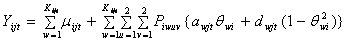Where:
mw jt   =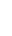the average of the phenotypic values of homozygous dominant and  homozygous recessive         genotypes of   wth   locus at jth environment and  tth replication within jth environment.

Kijt   =              the number of active loci  ith entry at jth environment and tth  replication within jth environment. The numbers of active loci vary among entries or genotypes because of hypostatic relation (interaction) among some genes.

Piwuv=  the frequency of AvAu genotype at wth locus in the ith  entry.

Following relation holds good for different populations:

Purelines: Piwu =0 or 1; Piwuv= 0 if u¹v
Mixtures: 0
£Piwu ³ 1;  Piwuv= 0 if u ¹ v
Hybrids: Piwu = 0 or 0.5 or 1;    Piwuv = 0 or 1 if u
¹ v
Synthetic:P2iwu = Piwuu; 2Piwu Piwv = Piwuv and P2iwv = Piwvv
In all cases, Piwu +Piwv = 1.0

awjt  =   the mean  of the difference between the phenotypic values of homozygous dominant and homozygous recessive genotype of  wth locus at jth environment and tth replication within jth environment . awjt is zero for all inactive loci. A hypostatic gene (allele) is considered inactive.

dwjt  =  the difference between the phenotype values of a heterozygous genotype of wth locus and its corresponding mwjt at the jth environment and tth replication within the jth   environment.

qwi  =  1, 0, -1 for homogygous dominant, heterozygote and homozygous  recessive genotype,   respectively, of wth locus in the ith  entry.

# GENERATING PERFORMANCE

Different populations: purelines and composites were generated as of Subedi (1996). The yield of a genotype is generated at two levels: a) first of all, the genotype is generated and then genetic constitution parameters (Ki, Ai , Di) are computed based on its genetic constitution, and b) these parameters are then multiplied by mj, aj and dj, respectively and added them together.

# GENERATING GEI

Different magnitudes and types of GEI are basically generated by changing the variance of distributions generating aj’s and dj’s.

AMMI MODEL

The basic linear model used in the analysis of GEI is of the form:

Yij= m  +gi  +Îj  + dij    : Where, (GXE)ij =dij       gi = genotypic effect of ith genotype, and Îj= environmental effect of jth environment and m=grand   mean = `Y..  In Additive Main Effects and Multiplicative Interactions  (AMMI) model (Shafii et al.,1992), the yield is partitioned as:

Yij= m  + gi + Îj + (l1 v1iu1j  + l2 v2iu2j +…)

lm is the singular value of the matrix ETE (square root of eigen value of PCA axis m), where E is the n x p matrix of residuals (dij) . The vmi and umj are corresponding normalized eigen vectors of matrix EET and ETE , respectively. Genotype PCA score and environment PCA score are expressed as unit vector times the square root of lm . The GEI sum of squares is divided into PCA axes where axis m is regarded as having n+p-1-2m degrees of freedom, where n and p are the number of genotypes and environments, respectively.

# COMPARING THE VAG MODEL WITH THE AMMI MODEL

Different criteria are used to compare these two models. First of all, variance of distribution generating aj’s of VAG model and l1 2 (sum of squares explained) by first component of AMMI model were regressed to find any relationship that exits between the models. Secondly, the GEI sum of squares explained by different components of the two models was compared.

# COMPUTING GENETIC ARCHITECTURE OF TEST GENOTYPES

Actual analysis of variance (ANOVA) of multi-environment tested performance of a set of genotypes is simulated and compared. If there exits a significant relationship among the different components of the two models between actual and simulated ANOVA, then these two ANOVA are declared similar. The Ai and Di effects if found similar to either first or second PCA scores warrant a situation where an approximate genetic constitution or architecture of the tested genotypes can be computed. For the sake of example, the rapeseed data of Shafii et al. (1992) was used in this study, to illustrate this point.

RESULTS AND DISCUSSION

The diagrams generated through varying magnitude and types of GEI are presented in Figure 1. Diagrams indicate basically two main points: a) the number of cross-over interactions are equal to or less than minimum of  (n,p):number of genotypes  or number of environments , and b) most of the variations in GEI is predicted by first two PCA  scores (PCA1 and PCA2).

The sum of squares of GEI predicted by different components of the VAG and the AMMI models are presented in Table 1. In some situations (first three cases), each component of both models predict almost equal magnitude of GEI, while rest of the cases, the same is not true. In the latter case also, the magnitude of GEI predicted by first two components (VAG2 vs AMMI2) are almost equal to themselves and to the  magnitude of actual GEI. This indicates the existence of following relationship:

VAG2 = ±l1 v1i u1j   ± l2 v2i u2j ±…= Yij - `Yi. - `Y.j + `Y..

It has been found (data not presented here) that the components of AMMI model may have similar or differing signs, throughout.

The relationship between the distribution generating aj’s and weighted eigen values (l12/np) is presented in Figure 2. The figure compares this relationship for two types of the populations: purelines and composites. This relationship should be viewed in the light of the last 3 sets of data in table 1. It indicates that there exists a significant relationship, although may not be 1:1, between the parameters of the two models.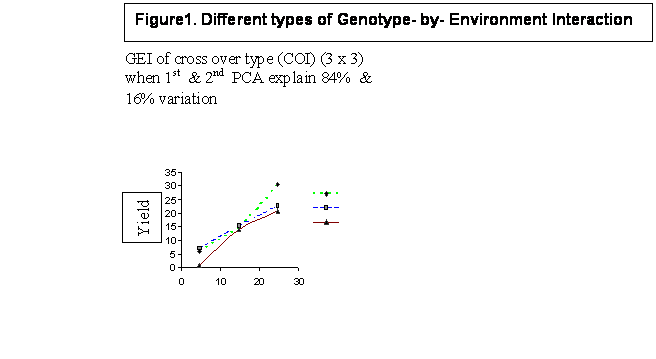Table 1. Comparison between different components of AMMI and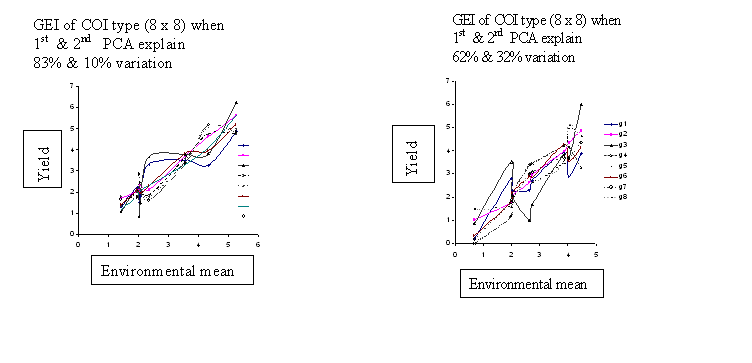VAG models for their predictability of sum of squares due to GEI

 AMMI1 VAG 1 AMMI 2 VAG 2 Actual Df (n=p) 12.33 12.77 19.27 19.76 20.38 49 19.41 19.47 31.16 30.03 37.18 49 42.61 51.68 63.1 68.1 67.0 49 11.06 1.38 13.51 12.13 12.93 4 19.89 7.75 19.76 17.86 19.91 4 11.44 0.61 12.81 11.51 12.26 4

AMMI 1 = l1v1i u1j                        AMMI 2 = l1v1i u1j + l2 v2i u2j

VAG 1 = (`Ai. - `A.) (aj-`a.)
VAG 2 = (
`Ai. - `A.) (aj-`a.) + (`Di. -`D.) (dj -`d.)

n = no. of genotypes           p = no. of environments